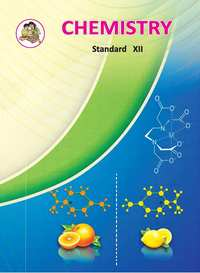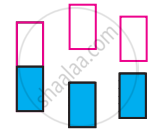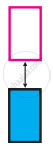Advertisement Remove all ads

# Balbharati solutions for Chemistry 12th Standard HSC for Maharashtra State Board chapter 1 - Solid State [Latest edition]

Advertisement Remove all ads

#### Chapters## Chapter 1: Solid State

Exercise
Exercise [Pages 25 - 27]

### Balbharati solutions for Chemistry 12th Standard HSC for Maharashtra State Board Chapter 1 Solid State Exercise [Pages 25 - 27]

Exercise | Q 1.1 | Page 25

Choose the most correct answer.

Molecular solids are _______.

• crystalline solids

• amorphous solids

• ionic solids

• metallic solids

Exercise | Q 1.2 | Page 25

Choose the most correct answer.

Which of the following is n-type semiconductor?

• Pure Si

• Si doped with As

• Si doped with Ga

• Ge doped with In

Exercise | Q 1.3 | Page 25

Choose the most correct answer.

In Frenkel defect, _______.

• electrical neutrality of the substance is changed

• density of the substance is changed

• both cation and anion are missing

• overall electrical neutrality is preserved

Exercise | Q 1.4 | Page 25

Choose the most correct answer.

In crystal lattice formed by bcc unit cell, the void volume is _______.

• 68 %

• 74 %

• 32 %

• 26 %

Exercise | Q 1.5 | Page 26

Choose the most correct answer.

The coordination number of atoms in bcc crystal lattice is _______.

• 2

• 4

• 6

• 8

Exercise | Q 1.6 | Page 26

Choose the most correct answer.

Which of the following is CORRECT?

• Four spheres are involved in the formation of a tetrahedral void.

• The centres of spheres in octahedral voids are at the apices of a regular tetrahedron.

• If the number of atoms is N, the number of octahedral voids is 2N.

• If the number of atoms is N/2, the number of tetrahedral voids is N.

Exercise | Q 1.7 | Page 26

Choose the most correct answer.

A compound forms hcp structure. Number of octahedral and tetrahedral voids in 0.5 mole of substance is respectively _______.

• 3.011 × 1023, 6.022 × 1023

• 6.022 × 1023, 3.011 × 1023

• 4.011 × 1023, 2.011 × 1023

• 6.011 × 1023, 12.022 × 1023

Exercise | Q 1.8 | Page 26

Choose the most correct answer.

Pb has fcc structure with edge length of unit cell 495 pm. Radius of Pb atom is _______.

• 205 pm

• 185 pm

• 260 pm

• 175 pm

Exercise | Q 2.01 | Page 26

Answer the following in one or two sentences.

What are the types of particles in each of the four main classes of crystalline solids?

Exercise | Q 2.02 | Page 26

Answer the following in one or two sentences.

Which of the three types of packing used by metals makes the most efficient use of space and which makes the least efficient use?

Exercise | Q 2.03 | Page 26

Answer the following in one or two sentences.

The following pictures show population of bands for materials having different electrical properties. Classify them as insulator, semiconductor or metal.Exercise | Q 2.04 | Page 26

Answer the following in one or two sentences.

What is the unit cell?

Exercise | Q 2.05 | Page 26

Answer the following in one or two sentences.

How does the electrical conductivity of a semiconductor change with temperature? Why?

Exercise | Q 2.06 | Page 26

Answer the following in one or two sentences.

The picture represents bands of MOs for Si. Label valence band, conduction band, and band gap.Exercise | Q 2.07 | Page 26

Answer the following in one or two sentences.

A solid is hard, brittle and electrically nonconductor. Its melt conducts electricity. What type of solid is it?

Exercise | Q 2.08 | Page 26

Answer the following in one or two sentences.

Mention two properties that are common to both hcp and ccp lattices.

Exercise | Q 2.09 | Page 26

Answer the following in one or two sentences.

Sketch a tetrahedral void.

Exercise | Q 2.1 | Page 26

Answer the following in one or two sentences.

What are ferromagnetic substances?

Exercise | Q 3.01 | Page 26

Answer the following in brief.

What are valence band and conduction band?

Exercise | Q 3.02 | Page 26

Answer the following in brief.

Distinguish between ionic solids and molecular solids

Exercise | Q 3.03 | Page 26

Answer the following in brief.

Calculate the number of atoms in fcc unit cell.

Exercise | Q 3.04 | Page 26

Answer the following in brief.

How are the spheres arranged in first layer of simple cubic close-packed structures? How are the successive layers of spheres placed above this layer?

Exercise | Q 3.05 | Page 27

Answer the following in brief.

Calculate the packing efficiency of metal crystal that has simple cubic structure.

Exercise | Q 3.06 | Page 27

Answer the following in brief.

What are paramagnetic substances? Give examples.

Exercise | Q 3.07 | Page 27

Answer the following in brief.

What are the consequences of Schottky defect?

Exercise | Q 3.08 | Page 27

Answer the following in brief.

Cesium chloride crystallizes in a cubic unit cell with Cl ions at the corners and a Cs+ ion in the centre of the cube. How many CsCl molecules are there in the unit cell?

Exercise | Q 3.09 | Page 27

Answer the following in brief.

Cu crystallizes in fcc unit cell with edge length of 495 pm. What is the radius of Cu atom?

Exercise | Q 3.1 | Page 27

Answer the following in brief.

Obtain the relationship between the density of a substance and the edge length of the unit cell.

Exercise | Q 4 | Page 27

The density of iridium is 22.4 g/cm3. The unit cell of iridium is fcc. Calculate the radius of iridium atom. Molar mass of iridium is 192.2 g/mol.

Exercise | Q 5 | Page 27

Aluminium crystallizes in a cubic close-packed structure with a unit cell edge length of 353.6 pm. What is the radius of Al atom? How many unit cells are there in 1.00 cm3 of Al?

Exercise | Q 6 | Page 27

In ionic crystalline solid atoms of element Y form hcp lattice. The atoms of element X occupy one-third of tetrahedral voids. What is the formula of the compound?

Exercise | Q 7 | Page 27

How are tetrahedral and octahedral voids formed?

Exercise | Q 8 | Page 27

Third layer of spheres is added to second layer so as to form hcp or ccp structure. What is the difference between the addition of third layer to form these close-packed structures?

Exercise | Q 9 | Page 27

An element with molar mass 27 g/mol forms a cubic unit cell with edge length of 405 pm. If the density of the element is 2.7 g/cm3, what is the nature of the cubic unit cell?

Exercise | Q 10 | Page 27

An element has a bcc structure with a unit cell edge length of 288 pm. How many unit cells and number of atoms are present in 200 g of the element? (Density of an element = 14.44 g/cm3 )

Exercise | Q 11 | Page 27

Distinguish with the help of diagrams metal conductors, insulators and semiconductors from each other.

Exercise | Q 12 | Page 27

What are n-type semiconductors? Why is the conductivity of doped n-type semiconductor higher than that of pure semiconductor? Explain with diagram.

Exercise | Q 13 | Page 27

Explain with diagram, Frenkel defect. What are the conditions for its formation? What is its effect on density and electrical neutrality of the crystal?

Exercise | Q 14 | Page 27

What is an impurity defect? What are its types? Explain the formation of vacancies through aliovalent impurity with example.

Advertisement Remove all ads

## Chapter 1: Solid State

Exercise## Balbharati solutions for Chemistry 12th Standard HSC for Maharashtra State Board chapter 1 - Solid State

Balbharati solutions for Chemistry 12th Standard HSC for Maharashtra State Board chapter 1 (Solid State) include all questions with solution and detail explanation. This will clear students doubts about any question and improve application skills while preparing for board exams. The detailed, step-by-step solutions will help you understand the concepts better and clear your confusions, if any. Shaalaa.com has the Maharashtra State Board Chemistry 12th Standard HSC for Maharashtra State Board solutions in a manner that help students grasp basic concepts better and faster.

Further, we at Shaalaa.com provide such solutions so that students can prepare for written exams. Balbharati textbook solutions can be a core help for self-study and acts as a perfect self-help guidance for students.

Concepts covered in Chemistry 12th Standard HSC for Maharashtra State Board chapter 1 Solid State are Solid State, Types of Solids, Classification of Crystalline Solids, Crystal Structure, Cubic System, Packing of Particles in Crystal Lattice, Packing Efficiency, Crystal Defects Or Imperfections, Electrical Properties of Solids, Magnetic Properties of Solids.

Using Balbharati 12th Board Exam solutions Solid State exercise by students are an easy way to prepare for the exams, as they involve solutions arranged chapter-wise also page wise. The questions involved in Balbharati Solutions are important questions that can be asked in the final exam. Maximum students of Maharashtra State Board 12th Board Exam prefer Balbharati Textbook Solutions to score more in exam.

Get the free view of chapter 1 Solid State 12th Board Exam extra questions for Chemistry 12th Standard HSC for Maharashtra State Board and can use Shaalaa.com to keep it handy for your exam preparation

Advertisement Remove all ads
Share
Notifications

View all notifications

Forgot password?